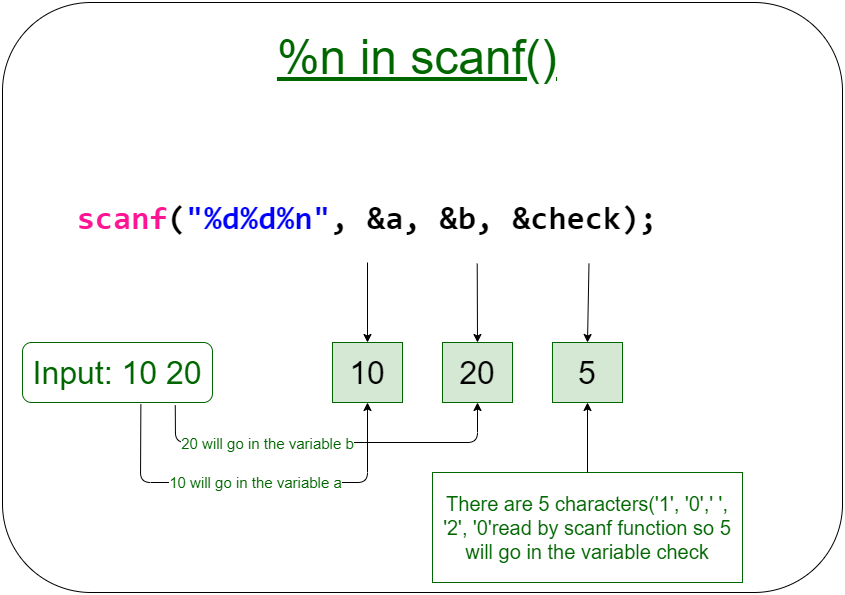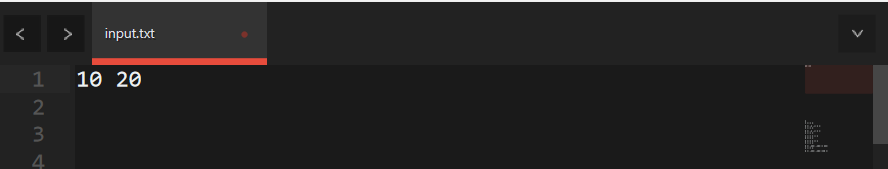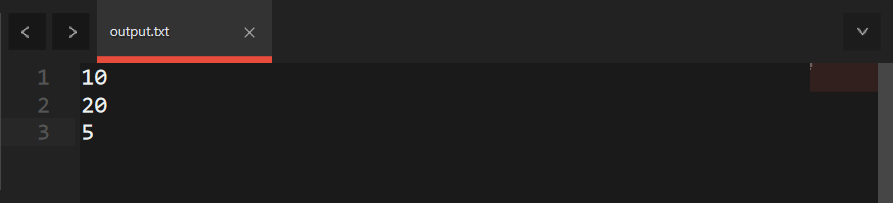# %n in scanf() in C with Example

• Last Updated : 19 Aug, 2020

In C, %n is a special format specifier. In the case of printf() function the %n assign the number of characters printed by printf(). When we use the %n specifier in scanf() it will assign the number of characters read by the scanf() function until it occurs.

Key points:

• It is an edit conversion code.
• The edit conversion code %[^\n] can be used as an alternative of gets.
• C supports this format specification with a scanf() function.
• This edit conversion code can be used to read a line containing characters like variables and even white spaces.
• In general scanf() function with format specification like %s and specification with the field width in the form of %ws can read-only strings till non-white space part.
• It means they cannot be used for reading a text containing more than one word, especially with white space.Syntax:

```scanf("%[^\n]", variable_name);
```

Below is the program to demonstrate the working of %n in scanf():

## C

 `// C++ to demonstrate %n in scanf() ` `#include ` ` `  `// Driver Code ` `int` `main() ` `{ ` `    ``int` `check; ` `    ``int` `a, b; ` ` `  `    ``// Input two variables ` `    ``scanf``(``"%d%d%n"``, &a, &b, &check); ` ` `  `    ``// Print value of a, b, and check ` `    ``printf``(``"%d\n%d\n%d"``, a, b, check); ` `    ``return` `0; ` `} `

Input:
Below is the input to the above code:Output:
Below is the output to the above code:Explanation:
scanf() function assigns the 10 and 20 in a and b respectively and 5 in variable check because there are 5 characters read by scanf function(including spaces).

My Personal Notes arrow_drop_up
Recommended Articles
Page :